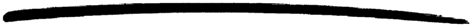# random

random

### MODULE SUMMARY

Pseudo random number generation

### DESCRIPTION

Random number generator. The method is attributed to B.A. Wichmann and I.D.Hill, in 'An efficient and portable pseudo-random number generator', Journal of Applied Statistics. AS183. 1982. Also Byte March 1987.

The current algorithm is a modification of the version attributed to Richard A O'Keefe in the standard Prolog library.

Every time a random number is requested, a state is used to calculate it, and a new state produced. The state can either be implicit (kept in the process dictionary) or be an explicit argument and return value. In this implementation, the state (the type ran()) consists of a tuple of three integers.

### EXPORTS

Seeds random number generation with default (fixed) values in the process dictionary, and returns the old state.

Types:

A1 = A2 = A3 = int()

Seeds random number generation with integer values in the process dictionary, and returns the old state.

One way of obtaining a seed is to use the BIF now/0:

```          ...
{A1,A2,A3} = now(),
random:seed(A1, A2, A3),
...

```

Returns the default state.

Returns a random float uniformly distributed between 0.0 and 1.0, updating the state in the process dictionary.

Types:

N = int()

Given an integer N >= 1, uniform/1 returns a random integer uniformly distributed between 1 and N, updating the state in the process dictionary.

Types:

State0 = State1 = ran()

Given a state, uniform_s/1returns a random float uniformly distributed between 0.0 and 1.0, and a new state.

Types:

N = int()
State0 = State1 = ran()

Given an integer N >= 1 and a state, uniform_s/2 returns a random integer uniformly distributed between 1 and N, and a new state.

### Note

Some of the functions use the process dictionary variable random_seed to remember the current seed.

If a process calls uniform/0 or uniform/1 without setting a seed first, seed/0 is called automatically.

stdlib 1.15
Copyright © 1991-2007 Ericsson AB